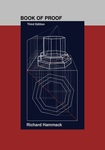## Open Access Textbooks#### Title

Book of Proof - Third Edition

#### Files

Download Full Text

#### Contributors

Richard Hammack - Author

#### Description

This is a book about how to prove theorems. Until this point in your education/ you may have regarded mathematics primarily as a computational discipline. You have learned to solve equations/ compute derivatives and integrals/ multiply matrices and find determinants; and you have seen how these things can answer practical questions about the real world. In this setting/ your primary goal in using mathematics has been to compute answers. But there is another approach to mathematics that is more theoretical than computational. In this approach/ the primary goal is to understand mathematical structures/ to prove mathematical statements/ and even to invent or discover new mathematical theorems and theories. The mathematical techniques and procedures that you have learned and used up until now have their origins in this theoretical side of mathematics. For example/ in computing the area under a curve/ you use the fundamental theorem of calculus. It is because this theorem is true that your answer is correct. However/ in your calculus class you were probably far more concerned with how that theorem could be applied than in understanding why it is true. But how do we know it is true? How can we convince ourselves or others of its validity? Questions of this nature belong to the theoretical realm of mathematics. This book is an introduction to that realm. This book will initiate you into an esoteric world. You will learn and apply the methods of thought that mathematicians use to verify theorems/explore mathematical truth and create new mathematical theories. This will prepare you for advanced mathematics courses/ for you will be better able to understand proofs/ write your own proofs and think critically and inquisitively about mathematics. This text has been used in classes at:Virginia Commonwealth University/ Lebanon Valley College/ University of California - San Diego/ Colorado State University/ Westminster College/ South Dakota State University/ PTEK College - Brunei/ Christian Brothers High School/ University of Texas Pan American/ Schola Europaea/ James Madison University/ Heriot-Watt University/ Prince of Songkla University/ Queen Mary University of London/ University of Nevada - Reno/ University of Georgia - Athens/ Saint Peter's University/ California State University/Bogaziçi University/ Pennsylvania State University/ University of Notre Dame

#### Subject 1

Mathematics - Pure

#### Subject 2

Mathematics - Pure

9.78E+12

Richard Hammack

#### Resources

Open Textbook Library

#### License

Attribution-NoDerivsCOinS